English

MQL4 BookOperatorsConditional Operator 'if - else'

# Conditional Operator 'if - else'

As a rule, if you write an application program, you need to code several solutions in one program. To solve these tasks, you can use the conditional operator 'if-else' in your code.

## Full Format

The full-format operator 'if-else' contains a heading that includes a condition, body 1, the key word 'else', and body 2. The bodies of the operator may consist of one or several operators; the bodies are enclosed in braces.

`   if ( Condition )                                     // Header of the operator and condition      {      Block 1 of operators                         // If the condition is true, then..      composing body 1                             //..the operators composing body 1 are executed      }else                                         // If the condition is false..      {      Block 2 of operators                        // ..then the operators..      composing body 2                             // ..of body 2 are executed      }`

## Format without 'else'

The operator 'if-else' can be used without its 'else' part. In this case, the operator 'if-else' contains its header that includes a condition, and body 1 that consists of one or several operators and enclosed in braces.

`   if ( Condition )                                  // Header of the operator and condition      {      Block 1 of operators                         // If the condition is true, then..      composing body 1                             //..the operators composing body 1 are executed      }`

## Format without Braces

If the body of the operator 'if-else' consists of only one operator, you can omit the enclosing braces.

`   if ( Condition )                                         // Header of the operator and condition      Operator;                                     // If the condition is true, then..                                                    // ..this operator is executed`

## Execution Rule for the Operator 'if-else'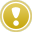If the condition of the operator 'if-else' is true, it passes the control to the first operator in body 1. After all operators in body 1 have been executed, it passes control to the operator that follows the operator 'if-else'. If the condition of the operator 'if-else' is false, then: - if there is the key word 'else' in the operator 'if-else', then it passes the control to the first operator in body 2. After all operators in body 2 have been executed, it passes control to the operator that follows the operator 'if-else'; - if there is no key word 'else' in the operator 'if-else', then it passes the control to the operator that follows the operator 'if-else'.

## Execution Examples of the Operator 'if-else'

Let us consider some examples that demonstrate how we can use the operator 'if-else'.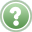Problem 9. Compose a program where the following conditions are realized: If the price for a symbol has grown and exceeded a certain value, the program must inform the trader about it; if it hasn't exceeded this value, the program mustn't perform any actions.

One of solutions for this problem may be, for example, as follows (onelevel.mq4):

`//---------------------------------------------------------------------------------------// onelevel.mq4// The code should be used for educational purpose only.//---------------------------------------------------------------------------------------int start()                                            // Special function 'start'  {   double   Level,                                              // Alert level   Price;                                              // Current price   Level=1.2753;                                       // Set the level   Price=Bid;                                          // Request price//---------------------------------------------------------------------------------------   if (Price>Level)                                    // Operator 'if' with a condition     {      Alert("The price has exceeded the preset level");// Message to the trader     }//---------------------------------------------------------------------------------------   return;                                             // Exit start()  }//---------------------------------------------------------------------------------------`

It should be noted, first of all, that the program is created as an Expert Advisor. This implies that the program will operate for quite a long time in order to display the necessary message on the screen as soon as the price exceeds the preset level. The program has only one special function, start(). At the start of the function, the variables are declared and commented. Then the price level is set in numeric values and the current price is requested.

The operator 'if-else' is used in the following lines of the program:

`//---------------------------------------------------------------------------------------   if (Price>Level)                                    // Operator 'if' with a condition     {      Alert("The price has exceeded the preset level");// Message to the trader     }//---------------------------------------------------------------------------------------`

As soon as the control in the executing program is passed to the operator 'if-else', the program will start to test its condition. Please note that the conditional test in the operator 'if-else' is the inherent property of this operator. This test cannot be omitted during execution of the operator 'if-else', it is a "raison d'etre" of this operator and will be performed, in all cases. Thereafter, according to the results of this test, the control will be passed to either the operator's body or outside it - to the operator that follows the closing brace.

In Fig. 37 below, you can see a block diagram representing a possible event sequence at execution of the operator 'if-else'.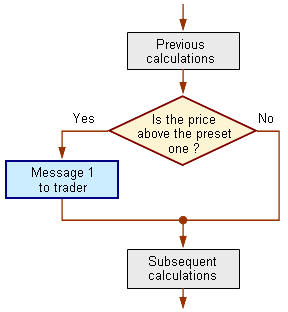Fig. 37. A block diagram for execution of the operator 'if-else' in program onelevel.mq4.

In this and all following diagrams, a diamond represents the conditional check. Arrows show the target components, to which the control will be passed after the current statement block has been executed (the term of statement block means a certain random set of operators adjacent to each other). Let's consider the diagram in more details.

The block of "Previous Calculations" (a gray box in the diagram) in program onelevel.mq4 includes the following:

`   double   Level,                                     // Alert level   Price;                                     // Current price   Level=1.2753;                              // Set the level   Price=Bid;                                 // Request price`

After the last operator in this block has been executed, the control is passed to the header of the operator 'if-else' where the condition of Does the price exceed the preset level? (the diamond box in the diagram, Fig. 37) is checked:

`   if (Price>Level)                           // Operator 'if' with a condition`

In other words, we can say that the program at this stage is groping for the answer to the following question: Is the statement enclosed in parentheses true? The statement itself sounds literally as it is written: The value of the variable Price exceeds that of the variable Level (the price exceeds the preset level). By the moment of checking this statement for being true or false, the program has already had the numeric values of variables Price and Level. The answer depends on the ratio between these values. If the price is below the preset level (the value of Price is less or equal to the value of Level), the statement is false; if the price exceeds this level, the statement is true.

Thus, where to pass the control after the conditional test depends on the current market situation (!). If the price for a symbol remains below the preset level (the answer is No, i.e., the statement is false), the control, according to the execution rule of the operator 'if-else', will be passed outside the operator, in this case, to the block named Subsequent Calculations, namely, to the line:

`   return;                                    // Exit start()`

As is easy to see, no message to the trader is given.

If the price for a symbol exceeds the level preset in the program (the answer is Yes, i.e., the statement is true), the control will be passed to the body of the operator 'if-else', namely, to the following lines:

`      {      Alert("The price has exceeded the preset level");// Message to the trader      }`

The execution the function Alert() will result in displaying on the screen a small box containing the following message:

 The price has exceeded the preset level

The function Alert() is the only operator in the body of the operator 'if-else', this is why after its execution, the operator 'if-else' is considered to be completely executed, and the control is passed to the operator that follows the operator 'if-else', i.e., to line:

`   return;                                    // Exit start()`

The execution of the operator 'return' results in that the function start() finishes its work, and the program switches to the tick-waiting mode. At a new tick (that also bears a new price for the symbol), the function start() will be executed again. So the message coded in the program will or won't be given to the trader depending on whether the new price exceeds or does not exceed the preset level.

The operators 'if-else' can be nested. In order to demonstrate how the nested operators can be used, let's consider the next example. The problem in it is a bit more sophisticated.Problem 10. Compose a program where the following conditions are realized: If the price has grown so that it exceeds a certain level 1, the program must inform the trader about it; if the price has fallen so that it becomes lower than a certain level 2, the program must inform the trader about it; however, the program mustn't perform any actions, in any other cases.

It is clear that, in order to solve this problem, we have to check the current price twice:

1. compare the price to level 1, and

2. compare the price to level 2.

## Solution 1 of Problem 10

Acting formally, we can compose the following solution algorithm: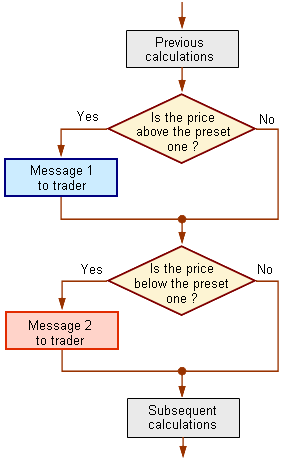Fig. 38. A block diagram of operators 'if-else' to be executed in program twolevel.mq4.

The program that realizes this algorithm can be as follows (twolevel.mq4):

`//-----------------------------------------------------------------------// twolevel.mq4// The code should be used for educational purpose onlyl.//-----------------------------------------------------------------------int start()                                 // Special function 'start'  {   double   Level_1,                                 // Alert level 1   Level_2,                                 // Alert level 2   Price;                                   // Current price   Level_1=1.2850;                          // Set level 1   Level_2=1.2800;                          // Set level 2   Price=Bid;                               // Request price//-----------------------------------------------------------------------   if (Price > Level_1)                     // Check level 1     {      Alert("The price is above level 1");  // Message to the trader     }//-----------------------------------------------------------------------   if (Price < Level_2)                     // Check level 2     {      Alert("The price is above level 2");  // Message to the trader     }//-----------------------------------------------------------------------   return;                                  // Exit start()  }//-----------------------------------------------------------------------`

As is easy to see, the code in program twolevel.mq4 is the extended version of program onelevel.mq4. If we had only one level of calculations before, we have two levels in this new program. Each level was defined numerically, and the program, to solve the stated problem, has two blocks that track the price behavior: Falls the price within the range of values limited by the preset levels or is it outside this range?

Let's give a brief description of the program execution.

After the preparatory calculations have been performed, the control is passed to the first operator 'if-else':

`//----------------------------------------------------------------------------------   if (Price > Level_1)                     // Checking the first level     {      Alert("The price is above level 1");  // Message to the trader     }//----------------------------------------------------------------------------------`

Whatever events take place at the execution of this operator (whether a message is given to the trader or not), after its execution the control will be passed to the next operator 'if-else':

`//----------------------------------------------------------------------------------   if (Price < Level_2)                     // Checking the second level     {      Alert("The price is below level 2");  // Message to the trader     }//----------------------------------------------------------------------------------`

The consecutive execution of both operators results in the possibility to perform both conditional tests and, finally, to solve the problem. While the program solves the task in full, this solution cannot be considered as absolutely correct. Please note a very important detail: The second conditional test will be executed regardless of the results obtained at testing in the first block. The second block will be executed, even in the case of the price exceeding the first level.

It should be noted here, that one of the important objectives pursued by the programmer when writing his or her programs is algorithm optimization. The above examples are just a demonstration, so they represent a short and, respectively, quickly executed program. Normally, a program intended to be used in practice is much larger. It can process the values of hundreds of variables, use multiple tests, each being executed within a certain amount of time. All this may result in the risk of that the duration of working of the special function start() may exceed the tick gaps. This will mean that some ticks may remain unprocessed. This is, of course, an extremely undesirable situation, and the programmer must do his or her best in order to prevent it. One of the techniques allowing to to reduce the time of the program execution is algorithm optimization.

Let's consider the latest example once again, this time in terms of resources saving. For example, what is the reason for asking "Is the price below the preset level?" in the second block, if the testing of the first block has already detected that the price is above the upper level? It is clear that, in this case, the price cannot be below the lower level, so there is no need to make a conditional test in the second block (nor to execute the set of operators in this block).

## Solution 2 of Problem 10

Taking the above reasoning into account, let's consider the following, optimized algorithm: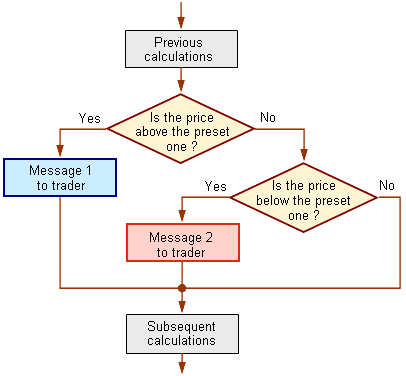Fig. 39. A Block diagram for execution of the operator 'if-else' in program twoleveloptim.mq4.

According the algorithm shown in the above block diagram, no redundant operations will be executed in the program. After the preparatory calculations, the program will test whether The price is above the preset level. If yes, the program displays the corresponding message to the trader and passes the control to Subsequent Calculations, whereas the second condition (Is the price below the preset level?) is not tested. Only if the price hasn't turned out to be above the upper level (the answer is No), the control is passed to the second block where the necessary conditional test is performed. If the price turns out to be below the lower level, the corresponding message will be displayed to the trader. If not, the control will be passed to the further calculations. It is obvious that, if the program works according this algorithm, no redundant actions are performed, which results in considerable saving of resources.

The programmed implementation of this algorithm implies the use of the nested operator 'if-else' (twoleveloptim.mq4):

`//-----------------------------------------------------------------------// twoleveloptim.mq4// The code should be used for educational purpose only.//-----------------------------------------------------------------------int start()                                  // Special function start()  {   double   Level_1,                                  // Alert level 1   Level_2,                                  // Alert level 2   Price;                                    // Current price   Level_1=1.2850;                           // Set level 1   Level_2=1.2800;                           // Set level 2   Price=Bid;                                // Request price//-----------------------------------------------------------------------   if (Price > Level_1)                      // Check level 1     {      Alert("The price is above level 1");   // Message to the trader     }   else     {      if (Price < Level_2)                   // Check level 2        {         Alert("The price is above level 2"); // Message to the trader        }     }//-----------------------------------------------------------------------   return;                                   // Exit start()  }//-----------------------------------------------------------------------`

Please note this block of calculations:

`//--------------------------------------------------------------------      if (Price > Level_1)                      // Check level 1     {      Alert("The price is above level 1");   // Message to the trader     }   else     {      if (Price < Level_2)                   // Check level 2        {         Alert("The price is above level 2"); // Message to the trader        }     }//--------------------------------------------------------------------`

The operator 'if-else', in which the second condition is tested, is a component of the first operator 'if-else' that tests the first condition. Nested operators are widely used in the programming practice. This is often reasonable and, in some cases, the only possible solution. Of course, instead of the function Alert(), real programs can use other functions or operators performing various useful actions.

A complex expression can also be used as a condition in the operator 'if-else'.Problem 11. Compose a program that realizes the following conditions: If the price falls within the preset range of values, no actions should be performed; if the price is outside this range, the program must inform the trader about it.

The statement of this problem is similar to that of Problem 10. The difference consists in that, in this case, we are not interested in the direction of the price movement - higher or lower than the predefined range. According to the problem situation, we have to know only the fact itself: Is the price within or outside the range? We can use the same text for the message to be displayed.

Here, like in the preceding solutions, it is necessary to check the price status as related to two levels - the upper one and the lower one. We have rejected the solution algorithm shown in Fig. 38 as non-efficient. So, according to the above reasoning, we can propose the following solution: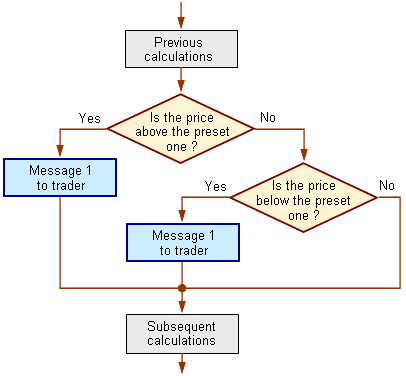Fig. 40. The block diagram of one of the solutions of problem 11.

However, there is no need to use this algorithm. The diagram demonstrates that the algorithm implies the usage of the same block of messages at different points in the program. The program lines will be repeated, notwithstanding that the execution of them will result in producing the same messages. In this case, it would be much more efficient to use the only one operator 'if-else' with a complex condition: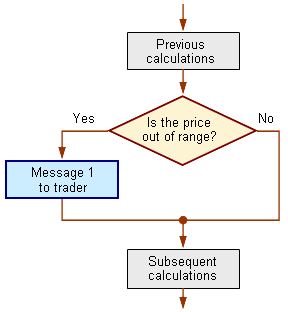Fig. 41. A block diagram for execution of the operator 'if-else' in program compoundcondition.mq4.

The code of the program that implements this algorithm is as follows (compoundcondition.mq4):

`//---------------------------------------------------------------------------------// compoundcondition.mq4// The code should be used for educational purpose only.//---------------------------------------------------------------------------------int start()                                           // Special function 'start'  {   double   Level_1,                                           // Alert level 1   Level_2,                                           // Alert level 2   Price;                                             // Current price   Level_1=1.2850;                                    // Set level 1   Level_2=1.2800;                                    // Set level 2   Price=Bid;                                         // Request price//--------------------------------------------------------------------------------   if (Price>Level_1 || Price<Level_2)               // Test the complex condition     {      Alert("The price is outside the preset range");// Message      }//--------------------------------------------------------------------------------   return;                                           // Exit start()  }//--------------------------------------------------------------------------------`

The key insight of this program solution is the use of complex condition in the operator 'if-else':

`if (Price>Level_1 || Price<Level_2)               // Test the complex condition  {   Alert("The price is outside the preset range");// Message   }`

Simply stated, this condition is as follows: If the value of variable Price exceeds that of variable Level_1, or the value of variable Price is less than that of variable Level_2, the program must execute the body of the operator 'if-else'. It is allowed to use logical operations (&&,|| and !) when composing the conditions of the operator 'if-else', they are widely used in the programming practice (see Boolean (Logical) Operations).

When solving other problems, you may need to compose even more complex conditions, which makes no question, too. Some expressions, including the nested ones, can be enclosed in parentheses. For example, you may use the following expression as a condition in the operator 'if-else':

`   if ( A>B && (B<=C || (N!=K && F>B+3)) )      // Example of a complex condition`

Thus, MQL4 opens up great opportunities to use the operators 'if-else': they can be nested, they can contain nested structures, they can use simple and complex test conditions, which results in the possibility to compose simple and complex programs with branching algorithms.Assignment OperatorCycle Operator 'while'